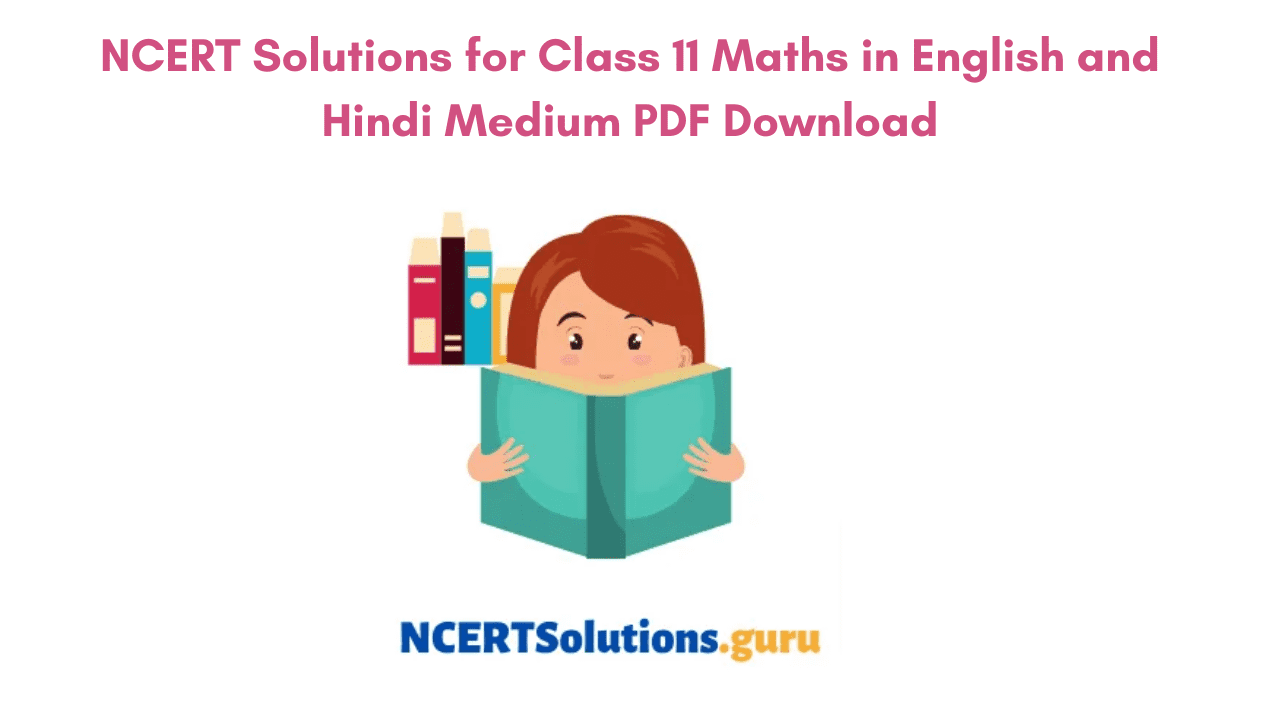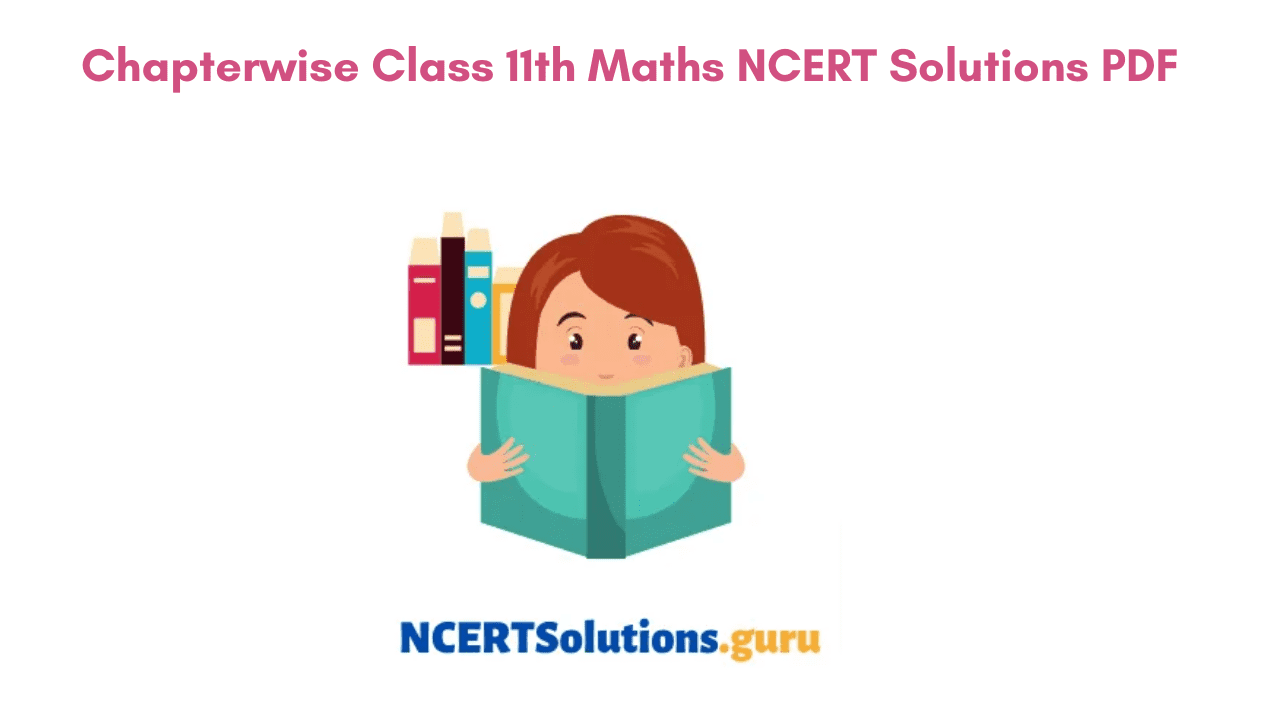# NCERT Solutions for Class 11 Maths in English and Hindi Medium | Chapterwise Class 11 Maths NCERT Solutions

NCERT Solutions for Class 11 Maths provided here are given by experts adhering to the Latest CBSE Syllabus Guidelines. NCERT Solutions for Class 11 Maths PDF has been created to simply all Class 11 Maths Problems in NCERT Maths Textbooks. Chapterwise NCERT Solutions of Class 11th Maths are organized systematically and covers the entire CBSE Class 11 Maths Syllabus. Download the 11th Class Maths NCERT Solutions PDF and develop comprehensive learning among you.

## NCERT Solutions for Class 11 Maths

The NCERT solutions for class 11 maths cover all the solutions of exercises given in chapters like a binomial theorem, trigonometric function, statistics, and many more chapters in the class 11 maths syllabus. The students just need to have access to the internet to go through the Class 11 Maths NCERT solutions. They can access these solutions anytime and from anywhere. Below is the overview of the chapters that we are providing for you to select the topics. You can choose from whichever medium you are comfortable with and learn the respective chapters easily.

### NCERT Solutions for Class 11 Maths English Medium

NCERT Solutions for Class 11 Maths Chapter 1

NCERT Solutions for Class 11 Maths Chapter 2

NCERT Solutions for Class 11 Maths Chapter 3

NCERT Solutions for Class 11 Maths Chapter 4

NCERT Solutions for Class 11 Maths Chapter 5

NCERT Solutions for Class 11 Maths Chapter 6

NCERT Solutions for Class 11 Maths Chapter 7

NCERT Solutions for Class 11 Maths Chapter 8

NCERT Solutions for Class 11 Maths Chapter 9

NCERT Solutions for Class 11 Maths Chapter 10

NCERT Solutions for Class 11 Maths Chapter 11

NCERT Solutions for Class 11 Maths Chapter 12

NCERT Solutions for Class 11 Maths Chapter 13

NCERT Solutions for Class 11 Maths Chapter 14

NCERT Solutions for Class 11 Maths Chapter 15

NCERT Solutions for Class 11 Maths Chapter 16

###NCERT Solutions of Class 11th Maths can be an extremely helpful resource for those preparing for the Class 11 Maths Exam. Our NCERT Solutions of Class 11 Maths can resolve all your doubts by providing you with shortcut tips, notes, study material, etc. Practice these NCERT Class 11th Maths Solutions PDF on a regular basis and score better marks in exams. Get Proper Guidance and have a thorough learning experience using the quick resources and have a quick review. Test your knowledge using the MCQ Questions for Class 11 Maths with Answers and improve accordingly.

#### NCERT Solutions of Class 11 Maths Chapter 1 Sets

The Chapter deals with concepts of sets like Empty Set, Finite, Infinite Sets, Equal Sets, Power Set, and Universal Set. Learn how to draw Venn Diagrams and get to know Union and Intersection of Sets, Difference of Sets, Complement of a Set and its Properties. There are 6 Exercises altogether in chapter 1 of sets.

#### NCERT Solutions for Class 11 Maths Chapter 2 Relations & Functions

Relations & Functions Chapter deals with concepts like Ordered Pairs, Cartesian Product of Sets, Number of Elements in Cartesian Product Product of 2 Finite Sets, Pictorial Diagrams, Domain, Co-domain, Range of a relation. Furthermore, it also deals with topics like pictorial representation of a function, domain, co-domain, and range of a function, modulus, signum, exponential, logarithmic functions, and their graphs. There are a total of 3 exercises and a Miscellaneous Exercise is present in this chapter.

#### NCERT Solutions for Class 11 Maths Chapter 3 Trigonometric Functions

Chapter 3 Trigonometric Functions describes positive and negative angles, methods for measuring angles in both radians and degrees, conversion from one measure to another. The chapter also deals with Trigonometric Functions Definition using Unit Circle, General Solution of Trigonometric Equations, Domain and Range of Trigonometric Functions and their Graphs. There are a total of 4 Exercises along with a Miscellaneous Exercise altogether in this chapter.

#### NCERT Solutions for Class 11 Maths Chapter 4 Principle of Mathematical Induction

The principle of Mathematical Induction deals with a number of topics covering the topics on proving induction, problems on mathematical induction principle, and applications are covered in this chapter.

#### Class 11 Maths NCERT Solutions Chapter 5 Complex Numbers and Quadratic Equations

Chapter 5 of Complex Numbers and Quadratic Equations tells students the algebraic properties of complex numbers, argand plane, polar representation of complex numbers, etc. It also includes information on the fundamental theorem of algebra statement, square root of a complex number, etc. Altogether there are 3 exercises and a miscellaneous exercise in this chapter.

#### NCERT Solutions of Class 11 Maths Chapter 6 Linear Inequalities

By the name itself, we can understand Class 11 Maths Chapter 6 deals with the topics of Linear Inequalities. It even discusses the algebraic solution of linear inequalities in one variable, linear inequalities in two variables, graphical representation on a number lines, etc. A total of 3 exercises and a miscellaneous exercise exist in this chapter 6.

#### NCERT Solutions of Class 11 Maths Chapter 7 Permutations and Combinations

Chapter 7 of Class 11 Maths Textbook deals with the definition of permutations, combinations, the fundamental principle of counting, factorial(n!), derivation of formulae, and their simple applications. All 4 Exercises and a Miscellaneous Exercise include all these topics in the chapter.

#### NCERT Solutions for Class 11 Maths Chapter 8 Binomial Theorem

In Chapter 8 Binomial Theorem, we will discuss the Binomial Theorem of Positive Integers and how it can be used to solve complex problems that are hard to solve using repeated multiplication. It elucidates the information on history, statement, and proof of Binomial Theorem for Positive Integer Indices. In addition topics like Pascal’s Triangle, General and Middle Term in Binomial Expansion, and their applications are explained. You can practice these questions from 2 exercises and a miscellaneous exercise in the chapter.

#### NCERT Solutions for Class 11 Maths Chapter 9 Sequences and Series

The Chapter discusses topics like Sequence and Series, Arithmetic Progression, Arithmetic Mean, Geometric Progression, General Terms of G.P, Sum of First n terms of a G.P, the relation between A.M. and G.M, formulae of special series and sums, etc. Altogether there are 4 exercises and a miscellaneous exercise that are designed to understand these concepts.

#### NCERT Solutions for Class 11 Maths Chapter 10 Straight Lines

Topics like various forms of equations of a line including the ones parallel to the axis, point-slope form, slope-intercept form, two-point form, Shifting of origin, the slope of a line, and angle between two lines, are discussed in this chapter. Along with these concepts you will come across the topics like the general equation of a line, equation of the family of lines passing through the point of intersection of two lines, etc. The 3 exercises and miscellaneous exercise present makes it easy for you to understand the topics.

#### NCERT Solutions of Class 11 Maths Chapter 11 Conic Sections

The concept of Conic Sections is discussed in detail here. Topics such as Section of a Cone like Circle, Ellipse, Parabola, Hyperbola, Straight Line, Point, Pair of Intersecting Lines are explained. In addition, standard equations, simple properties of circle, ellipse, hyperbola, parabola are discussed. A total of 4 exercises, and a miscellaneous exercise present in the chapter covers these topics.

#### NCERT Solutions for Class 11 Maths Chapter 12 Introduction to Three-dimensional Geometry

Students of Class 11 Maths will get to know the basic concepts of geometry in three-dimensional space here. You will get acquainted with topics such as coordinate planes, coordinate axes in three dimensions, coordinates of a point, distance between two points, and section formula. The 3 exercises present and a miscellaneous exercise will help students understand the concepts better. Mis

#### NCERT Solutions for Class 11 Maths Chapter 13 Limits and Derivatives

Limits and Derivatives Chapter prevailing will introduce you to Calculus a branch of Maths. Get an intrusive idea on derivatives by referring to this chapter. Know Definition of a Limit, Some Algebra of Limits, Derivative Definition, Algebra of Derivatives. The 2 exercises and a miscellaneous exercise present covers all these topics in the Limits and Derivatives Chapter.

#### NCERT Solutions of Class 11 Maths Chapter 14 Mathematical Reasoning

Chapter 14 deals with basic ideas of Mathematical Reasoning in the context of Mathematics. This Chapter includes fundamentals of deductive reasoning, mathematically acceptable statements connecting words/phrases, and understanding “if and only if”, “implies”, “and/or”, “implied by”, “and”, “or”, “there exists”, validating statements involving connecting words. 5 Exercises along with a Miscellaneous Exercise makes it easy for you to understand the concept of Mathematical Reasoning in a better way.

#### NCERT Solutions for Class 11 Maths Chapter 15 Statistics

Statistics is dealing with data collected for specific purposes. In this chapter, you will come across the important measures of dispersion and methods of ungrouped and grouped data. Furthermore, it includes topics like dispersion, range, mean deviation, variance, standard deviation, analysis of frequency distribution with equal means and different variances, etc. 3 Exercises and Additional Exercises present will make it easy for you to understand these topics.

#### NCERT Solutions for Class 11 Maths Chapter 16 Probability

Probability is a chapter that measures the uncertainty of various phenomena or chances of occurrence of an event. Get to know the concepts like random experiments outcomes, sample spaces, Events, Occurrence of Events, exhaustive events, mutually exclusive events, etc. Furthermore, the chapter even discusses info on Axiomatic (set theoretic) probability, connections with other theories learned in earlier classes. The chapter includes 3 exercises along with a miscellaneous exercise.### CBSE Class 11 Unit Wise Weightage of First Term

 No. Units Marks I Sets and Functions 11 II Algebra 13 III Coordinate Geometry 06 IV Calculus 04 V Statistics and Probability 06 Total 40 Internal Assessment 10 Total 50

### Class 11 Unit Wise Weighatge of Second Term

 Unit Unit Name Marks I Sets and Functions (Cont.) 08 II Algebra (Cont.) 11 III Coordinate Geometry (Cont.) 09 IV Calculus (Cont.) 06 V Statistics and Probability (Cont.) 06 Total 40 Internal Assessment 10 Total 50

### FAQs on NCERT Maths Book Class 11 Solutions PDF

1. Which is the best site for NCERT Solutions Class 11 Maths?

NCERTSolutions.guru is a trusted portal that provides the NCERT Solutions of Class 11 Maths in an efficient way.

2. What are the Best Books for Maths of Class 11 CBSE?

As far as my knowledge is concerned NCERT Books can be great as most of the questions asked in exams are framed from these books. Practice the concepts using the NCERT Exemplars and enhance your skills.

3. Is NCERT enough to have command over Class 11 Maths?

Yes, NCERT Solutions can be more than enough to have command over Class 11 Maths. You can crack board or competitive exams quite easily taking help from them.

### Conclusion

Hope the knowledge shared regarding the NCERT Solutions of Class 11 Maths has been helpful to the possible extent. If you have any queries don’t hesitate to ask us through the comment section so that we can respond to you. Keep in touch with us for the latest updates on NCERT Books, Solutions, Previous Papers, etc.

error: Content is protected !!